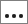# Define a Categorical Input Variable and Set the Allowable Values

A categorical variable is an input variable that can take values from a non-orderable finite list of values, for example x = red, green, blue.

This example assumes that you already have an input variable created.
1. In the Define Input Variables step, click the Modes tab.
2. In the Data Type column for the input variable you are defining, select String.
3. In the Mode column, select Categorical.
Note: The Values cell turns orange, which indicates you can edit its values.
4. In the Values cell, click.
5. In the dialog, define the categorical values.
6. Click Apply.
7. Click OK.